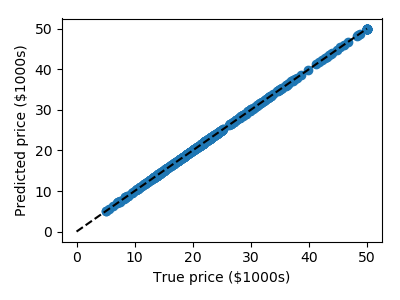# 3.6.10.1. Measuring Decision Tree performance¶

Demonstrates overfit when testing on train set.

Get the data

```from sklearn.datasets import load_boston
```

Train and test a model

```from sklearn.tree import DecisionTreeRegressor
clf = DecisionTreeRegressor().fit(data.data, data.target)

predicted = clf.predict(data.data)
expected = data.target
```

Plot predicted as a function of expected

```from matplotlib import pyplot as plt
plt.figure(figsize=(4, 3))
plt.scatter(expected, predicted)
plt.plot([0, 50], [0, 50], '--k')
plt.axis('tight')
plt.xlabel('True price (\$1000s)')
plt.ylabel('Predicted price (\$1000s)')
plt.tight_layout()
```Pretty much no errors!

This is too good to be true: we are testing the model on the train data, which is not a mesure of generalization.

The results are not valid

Total running time of the script: ( 0 minutes 0.065 seconds)

Gallery generated by Sphinx-Gallery